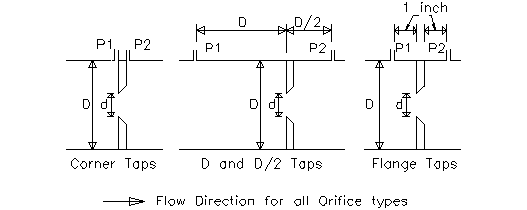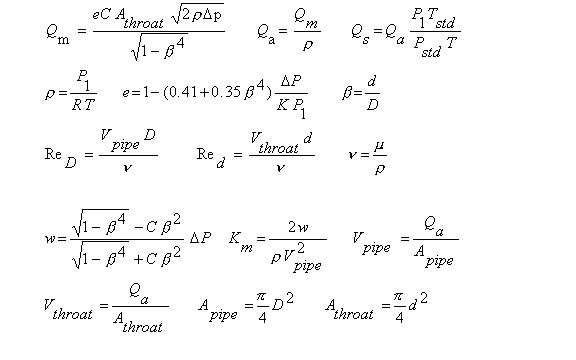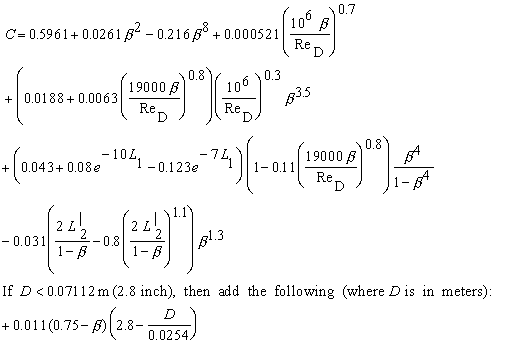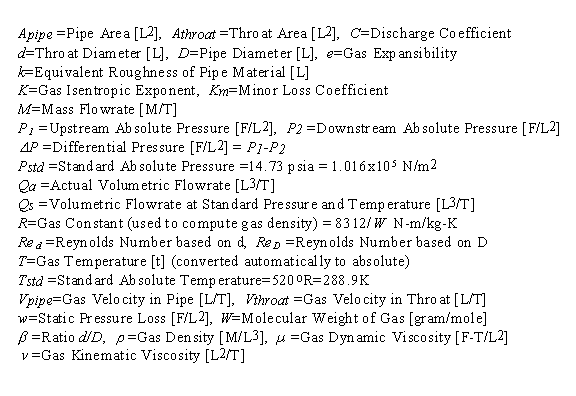Large Diameter Orifice Flow Meter Calculation for Gas Flow Rate Measurement

For pipe diameter ≥ 5 cm. Compute flow rate, orifice diameter, or differential pressure. Equations: ISO 5167

Register to enable "Calculate" button in flow rate measurement.

 Mass Flow, M (kg/s): Actual Vol Flow, Qa (m3/s): Solve for: Std Vol Flow, Qs (std m3/s): Flow Rate Differential Pressure. (M known) Differential Pressure. (Qa known) Differential Pressure. (Qs known) Throat Diameter. (M known) Throat Diameter. (Qa known) Throat Diameter. (Qs known) Upstream Abs Pressure, P1 (Pa): Differential Pressure, Δp (Pa): Select Meter Type and Gas: Pressure Ratio, P2/P1: Air (enter P1, T) Carbon dioxide (enter P1, T) Hydrogen (enter P1, T) Methane, Natural Gas (enter P1, T) Nitrogen (enter P1, T) Oxygen (enter P1, T) User enters P1, density, viscosity, K Throat Diameter, d (m): Corner Pressure Taps D and D/2 Pressure Taps Flange Pressure Taps Pipe Diameter, D (m): Ratio, d/D: Select Units for each Variable: Gas Temperature, T (K): Mass Flow in slug/s Mass Flow in lb (mass)/s Mass Flow in lb (mass)/hr Mass Flow in kg/s Density (kg/m3): Qa and Qs in ft3/s or cfs Qa and Qs in ft3/min or cfm Qa and Qs in ft3/hr Qa and Qs in ft3/day Qa and Qs in m3/s Qa and Qs in m3/min Qa and Qs in m3/hr Qa and Qs in m3/day Dynamic Viscosity (N-s/m2): P1 in psi P1 in inch H2O (60F) P1 in inch Mercury (60F) P1 in millibars P1 in N/m2 or Pa P1 in mm Mercury (0C) Isentropic Exponent, K: Diff P and P Loss in psi Diff P and P Loss in inch H2O (60F) Diff P, P Loss in inch Mercury (60F) Diff P and P Loss in millibars Diff P and P Loss in N/m2 or Pa Diff P and P Loss in mm Mercury (0C) Gas Expansibility, e: Diameters in inches Diameters in feet Diameters in cm Diameters in meters Discharge Coefficient, C: Temperature in Fahrenheit, F Temperature in Rankine, R Temperature in Celsius, C Temperature in Kelvin, K Reynolds No. based on d, Red: Density in slug/ft3 Density in lb(mass)/ft3 Density in kg/m3 Density in g/cm3 Reynolds No. based on D, ReD: Viscosity in lb-s/ft2, slug/ft-s Viscosity in N-s/m2, kg/m-s Viscosity in poise, P Viscosity in centiPoise, cP Pressure Loss, w (Pa): Velocity in ft/s; Area in ft2 Velocity in m/s; Area in m2 Minor Loss Coefficient, Km: © 2015 LMNO Engineering, Throat Velocity, Vthroat (m/s): Research, and Software, Ltd. Pipe Velocity, Vpipe (m/s): http://www.LMNOeng.com Throat Area, Athroat (m2): Pipe Area, Apipe (m2):

Units in large bore orifice flow meter for gas flow rate measurement calculation: C=degrees Celsius, cm=centimeter, cP=centipoise, cSt=centistoke, F=degrees Fahrenheit, cfm=cubic feet per minute, cfs=cubic feet per second, ft=foot, g=gram, hr=hour, in=inch, K=degrees Kelvin, kg=kilogram, lb=pound, m=meters, mbar=millibar, min=minute, mm=millimeter, N=Newton, Pa=Pascal, psi=pound per square inch, R=degrees Rankine, s=second, scfm=standard cfm, std=standard.

Types of Pressure Taps for OrificesTopics on flow rate measurement:  Introduction    Equations    Discharge Coefficient    Variables     Validity and Discussion    Error Messages    References

Introduction to Flow Rate Measurement
Orifice flow meters are used to determine a liquid or gas flow rate by measuring the differential pressure (P1 - P2) across the orifice plate.  Orifice flow rate measurement meters are typically less expensive to install and manufacture than the other commonly used differential pressure flow rate meters; however, nozzle and venturi flow rate meters have the advantage of lower pressure drops. Equations for orifice flow rate measurement meters have the advantage of no Reynolds Number upper limit for validity.

An orifice flow rate measurement meter is typically installed between flanges connecting two pipe sections (flanges are not shown in the above drawings). The three standard pressure tapping arrangements are shown in the drawings; the location of the pressure taps affects the discharge coefficient somewhat. Flange pressure taps penetrate the flange and are at a standard distance of 1 inch (2.54 cm) from either side of the orifice. For corner taps or D and D/2 taps, the pressure tap locations are as shown.

Orifices are typically less than 0.05D thick. For exact geometry and specifications for orifices, see ISO (1991) or ASME (1971). The ASME and ISO have been working on guidelines for orifices for flow rate measurement since the early 1900s. The organizations have the most confidence in orifice accuracy when the Reynolds number exceeds 105, though Reynolds numbers as low as 4x103 are valid for certain d/D ratios as discussed below. The flow rate measurement calculation above is for flow rate measurement of gases. For liquid flow through orifices, please visit our orifice flow rate measurement calculations for liquids: Pipe diameter < 5 cm or pipe diameter > 5 cm. Gas flow rate measurement calculations include an expansibility factor (e), which is not present in the liquid flow rate measurement calculation. The expansibility factor accounts for the effect of pressure change on gas density as gas flows through the orifice. LMNO Engineering also has an orifice calculation for gas flow rate measurement in pipes less than 5 cm diameter.

Equations for Flow Rate Measurement                             Top of Page
The flow rate measurement calculations on this page are for orifices carrying a gas as described in ISO (1991 and 1998).Discharge Coefficient (ISO, 1998)Corner Pressure Taps:  L1 = L'2 = 0
D and D/2 Pressure Taps:  L1 = 1  and  L'2 = 0.47
Flange Pressure Taps:  L1 = L'2 = 0.0254/D  where D is in meters

Variables for Flow Rate Measurement                             Top of Page
Dimensions: F=Force, L=Length, M=Mass, T=Time, t=temperatureValidity and Discussion for Gas Flow Rate Measurement                             Top of Page
Discharge Coefficient for Gas Flow Rate Measurement:
For all types of pressure taps:  d ≥ 1.25 cm,  5 cm ≤ D ≤ 1 m,  0.1 ≤ d/D ≤ 0.75

For Corner Pressure Taps or D and D/2 Pressure Taps:
ReD ≥ 4000  for  0.1 ≤ d/D ≤ 0.5   and  ReD ≥ 16,000(d/D )2  for  d/D>0.5

For Flange Pressure Taps:  ReD ≥ 4000  and  ReD ≥ 170,000 D (d/D )2  where D is in meters

The calculation does not provide results if these values are out of range.

Expansibility:
The equation shown above for expansibility, e, is valid for P2/P1 ≥ 0.75.  Our flow rate measurement calculation gives a warning message if  P2/P1 < 0.75, but still computes answers.

Built-in Properties for Certain Gases in Flow Rate Measurement Calculation:
To provide ease of use, our flow rate measurement calculation has properties of some gases built-in to the calculation. The user can select Air, Carbon dioxide, Hydrogen, Methane (natural gas), Nitrogen, or Oxygen. The density is automatically computed using the ideal gas law based on the upstream pressure and temperature entered into the gas flow rate measurement calculation. The dynamic viscosity is a function of temperature and uses the methodology shown on our Gas Viscosity page. The isentropic exponent, K, is based on the specific heat ratio. For methane, the dynamic viscosity value shown in the flow rate measurement calculation is valid for 0 oF < T < 1000 oF. If T<0 oF, then the viscosity value shown and used in the flow rate measurement computation is the viscosity at 0 oF. If T>1000 oF, then the viscosity value shown and used in the flow rate measurement computation is the viscosity at 1000 oF (0 oF is -17.8 oC and 1000 oF is 537.8 oC). For all other gases shown in the drop-down menu, there is no temperature limitation on the validity of the viscosity. Dynamic viscosity is essentially independent of pressure.

If you know that your density, viscosity, or isentropic exponent is significantly different than the value shown in the flow rate measurement calculation, then you can select "User enters P1, density, viscosity, K" and enter these values manually. Also, if the gas is not listed in our drop-down menu, then you can select "User enters P1, density, viscosity, K" and enter these values manually. K must be > 1. Additionally, values for K can be found in Weast (1985, p. F-11), Perry and Green (1984, p. 3-144), and other sources.

Note that our flow rate measurement calculation prior to February 2003 included helium as a gas in the drop-down gas menu, and the viscosities for the gases were set at 20 oC. Now, the viscosity variation with temperature is included, but helium was removed because it doesn't have a simple viscosity relationship with temperature.

ISO Pipe Roughness Recommendation:
ISO recommends that in general  k/D ≤ 3.8x10-4   for Corner Taps and  k/D ≤ 10-3 for Flange or D and D/2 pressure taps.  k is the pipe roughness. Our flow rate measurement calculation does not check this.

Pressure Loss:
w is the static pressure loss occurring from a distance of approximately D upstream of the orifice to a distance of approximately 6D downstream of the orifice. It is not the same as differential pressure. Differential pressure is measured at the exact locations specified in ISO (1991) (shown in the above figures).

Minor Loss Coefficient:
Km is computed to allow you to design pipe systems with orifices and incorporate their head loss. Head loss is computed as h=KmV2pipe/2g.

Standard Volumetric Flow Rate:
Standard volumetric flow rate, Qs, is the volumetric flow rate computed at standard pressure and temperature, Pstd and Tstd (shown above in variables). Actual flow rate, Qa, is computed at the gas's actual pressure and temperature. Qs is useful to users who need to compute (or input) standard flow rate; often pump curves and flow measurement devices provide standard, rather than actual, flow rate. The advantage of using standard flow instead of actual flow is that the same device (or pump curve) can be used for a gas at various temperatures and pressures without re-calibrating for an infinite range of actual pressures and temperatures. The user can easily convert standard to actual flow rate if the actual temperature and pressure of the gas are known; our flow rate measurement calculation does this automatically.

Error Messages given by flow rate measurement calculation                             Top of Page
"P2/P1<0.75. Out of range". The equation for expansibility, e, is only valid for P2/P1≥0.75. This is a just a warning message; all variables are computed.
For the following error messages, only some variables are computed by the flow rate measurement calculation. For example if throat diameter (d) is to be computed, then pressure ratio, expansibility, pipe area, pipe velocity, ReD, and some other variables will be computed and shown. However, if ReD is out of range for C to be valid, then C and d (and anything depending on d - such as throat area and throat velocity) will not be computed. If an error message is shown and you think your input is correct, be sure to check that you have selected the correct units for your entries.
"Infeasible input". While none of the inputs alone are out of range, they collectively result in a physically infeasible situation.
"P1 and T (abs) must be >0". Absolute pressure or absolute temperature was entered as zero or negative. If temperature was entered in C or F, it was internally converted to absolute temperature.
"P1,D, T(abs), Dens, Visc must be >0". Pipe diameter, density, viscosity, absolute pressure, and/or absolute temperature was entered as zero or negative.
"K must be >1". Isentropic exponent was entered as ≤ 1.
"d/D must be <1". Orifice diameter cannot exceed pipe diameter in the flow rate measurement calculation.
"M or Q, and d must be >0". Mass flow rate, volumetric flow rates, and/or orifice diameter were entered into the flow rate measurement calculation as zero or negative.
"d, D, d/D, or ReD out of range". At least one of these variables is out of the range of validity for the discharge coefficient.
"Δp and d must be >0". Cannot enter pressure difference or d ≤0.
"Δp must be < P1". Differential pressure cannot exceed P1; this would cause P2(absolute) to be <0 which is impossible.
"d/D out of range""D out of range", "d out of range" These must be in the range of validity shown above.
"M or Q, and Δp must be >0". Mass flow rate, volumetric flow rates, and/or differential pressure were entered as zero or negative in the flow rate measurement calculation.
"ReD out of range". ReD must be in range of validity shown above.
"ReD will be too small", "ReD will be out of range", "d/D will be out of range", "d will be <0.0125 m", "d/D will be < 0.1", "d/D will be > 0.75". Input values result in variables that will be out of the range of validity.
Try the simpler orifice flow rate calculation on our Bernoulli page if your parameters are out of range. It is not as accurate, but won't give "parameter out of range" error messages.

References for Flow Rate Measurement                             Top of Page
American Society of Mechanical Engineers (ASME). 1971. Fluid meters: Their theory and application. Edited by H. S. Bean. 6ed. Report of ASME Research Committee on Fluid Meters.

International Organization of Standards (ISO 5167-1). 1991. Measurement of fluid flow by means of pressure differential devices, Part 1: Orifice plates, nozzles, and venturi tubes inserted in circular cross-section conduits running full. Reference number: ISO 5167-1:1991(E).

International Organization of Standards (ISO 5167-1) Amendment 1. 1998. Measurement of fluid flow by means of pressure differential devices, Part 1: Orifice plates, nozzles, and venturi tubes inserted in circular cross-section conduits running full. Reference number: ISO 5167-1:1991/Amd.1:1998(E).

Perry, R. H. and D. W. Green (editors). 1984. Perry's Chemical Engineers' Handbook. McGraw-Hill Book Co. 6th ed.

Weast, R. C. (editor). 1985. CRC Handbook of Chemistry and Physics. Chemical Rubber Company. 65th ed.

LMNO Engineering, Research, and Software, Ltd.
7860 Angel Ridge Rd.   Athens, Ohio  45701  USA   Phone: (740) 707-2614
LMNO@LMNOeng.com    http://www.LMNOeng.com

To:

Other Flow Meter Calculations using standard methodologies:

Orifice for gases (D<5 cm)

Orifice for liquids (D<5cm)

Orifice for liquids (D>5cm)

Nozzle for liquids

Venturi for liquids

Simpler orifice calculation (not as accurate but won't give "parameter out of range" messages):

Bernoulli calculation

Pressure Relief Valve

Register